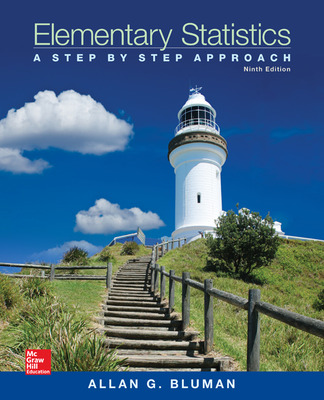# Elementary Statistics: A Step By Step Approach

9th Edition
By Allan Bluman
ISBN10: 0073534986
ISBN13: 9780073534985

## Purchase Options

Students, we’re committed to providing you with high-value course solutions backed by great service and a team that cares about your success. See tabs below to explore options and pricing. Don't forget, we accept financial aid and scholarship funds in the form of credit or debit cards.

### Hardcopy

• Bound book containing the complete text
• Full color
• Hardcover or softcover

### Hardcopy

• Bound book containing the complete text
• Full color
• Hardcover or softcover

The estimated amount of time this product will be on the market is based on a number of factors, including faculty input to instructional design and the prior revision cycle and updates to academic research-which typically results in a revision cycle ranging from every two to four years for this product. Pricing subject to change at any time.

### 1 The Nature of Probability and Statistics

1.1 Descriptive and Inferential Statistics
1.2 Variables and Types of Data
1.3 Data Collection and Sampling Techniques
1.4 Observational and Experimental Studies
1.5 Uses and Misuses of Statistics
1.6 Computers and Calculators

### 2 Frequency Distributions and Graphs

2.1 Organizing Data
2.2 Histograms, Frequency Polygons, and Ogives
2.3 Other Types of Graphs

### 3 Data Description

3.1 Measures of Central Tendency
3.2 Measures of Variation
3.3 Measures of Position
3.4 Exploratory Data Analysis

### 4 Probability and Counting Rules

4.1 Exploratory Data Analysis
4.2 The Addition Rules for Probability
4.3 The Multiplication Rules and Conditional Probability
4.4 Counting Rules
4.5 Probability and Counting Rules

### 5 Discrete Probability Distributions

5.1 Probability Distributions
5.2 Mean, Variance, Standard Deviation, and Expectation
5.3 The Binomial Distribution
5.4 Other Types of Distributions (Optional)

### 6 The Normal Distribution

6.1 Normal Distributions
6.2 Applications of the Normal Distribution
6.3 The Central Limit Theorem
6.4 The Normal Approximation to the Binomial Distribution

### 7 Confidence Intervals and Sample Size

7.1 Confidence Intervals for the Mean When σ Is Known
7.2 Confidence Intervals for the Mean When σ Is Unknown
7.3 Confidence Intervals and Sample Size for Proportions
7.4 Confidence Intervals for Variances and Standard Deviations

### 8 Hypothesis Testing

8.1 Steps in Hypothesis Testing—Traditional Method
8.2 z Test for a Mean
8.3 t Test for a Mean
8.4 z Test for a Proportion
8.5 χ2 Test for a Variance or Standard Deviation
8.6 Additional Topics Regarding Hypothesis Testing

### 9 Testing the Difference Between Two Means, Two Variances, and Two Proportions

9.1 Testing the Difference Between Two Means: Using the z Test
9.2 Testing the Difference Between Two Means of Independent Samples: Using the t Test
9.3 Testing the Difference Between Two Means: Dependent Samples
9.4 Testing the Difference Between Proportions 9.5 Testing the Difference Between Two Variances

### 10 Correlation and Regression

10.1 Scatter Plots and Correlation
10.2 Regression
10.3 Coefficient of Determination and Standard Error of the Estimate
10.4 Multiple Regression (Optional)

### 11 Other Chi-Square Tests

11.1 Test for Goodness of Fit
11.2 Tests Using Contingency Tables

### 12 Analysis of Variance

12.1 One-Way Analysis of Variance
12.2 The Scheffé Test and the Tukey Test
12.3 Two-Way Analysis of Variance

### 13 Nonparametric Statistics

13.1 Advantages and Disadvantages of Nonparametric Methods
13.2 The Sign Test
13.3 The Wilcoxon Rank Sum Test
13.4 The Wilcoxon Signed-Rank Test
13.5 The Kruskal-Wallis Test
13.6 The Spearman Rank Correlation Coefficient and the Runs Test

### 14 Sampling and Simulation

14.1 Common Sampling Techniques
14.2 Surveys and Questionnaire Design
14.3 Simulation Techniques and the Monte Carlo Method

### Appendices

Appendix A: Tables
Appendix B: Data Bank
Appendix C: Glossary
Appendix D: Photos Credits
Appendix E: Selected Answers

### Additional Topics Onlne

Algebra Review
Writing the Research Report
Bayes' Theorem
Alternate Approach to the Standard Normal Distribution
Bibliography